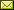## A Formalization of Finite Group Theory

 David M. Russinoff

 Previous formulations of group theory in ACL2 and Nqthm, based on either "encapsulate" or "defn-sk", have been limited by their failure to provide a path to proof by induction on the order of a group, which is required for most interesting results in this domain beyond Lagrange's Theorem (asserting the divisibility of the order of a group by that of a subgroup). We describe an alternative approach to finite group theory that remedies this deficiency, based on an explicit representation of a group as an operation table. We define a "defgroup" macro for generating parametrized families of groups, which we apply to the additive and multiplicative groups of integers modulo n, the symmetric groups, arbitrary quotient groups, and cyclic subgroups. In addition to a proof of Lagrange's Theorem, we present an inductive proof of the abelian case of a theorem of Cauchy: If the order of a group G is divisible by a prime p, then G has an element of order p.

In Rob Sumners and Cuong Chau: Proceedings Seventeenth International Workshop on the ACL2 Theorem Prover and its Applications (ACL2 2022), Austin, Texas, USA, 26th-27th May 2022, Electronic Proceedings in Theoretical Computer Science 359, pp. 99–115.
Published: 24th May 2022.

 ArXived at: http://dx.doi.org/10.4204/EPTCS.359.10 bibtex PDF
References in reconstructed bibtex, XML and HTML format (approximated).
 Comments and questions to:eptcs@eptcs.org For website issues:webmaster@eptcs.org